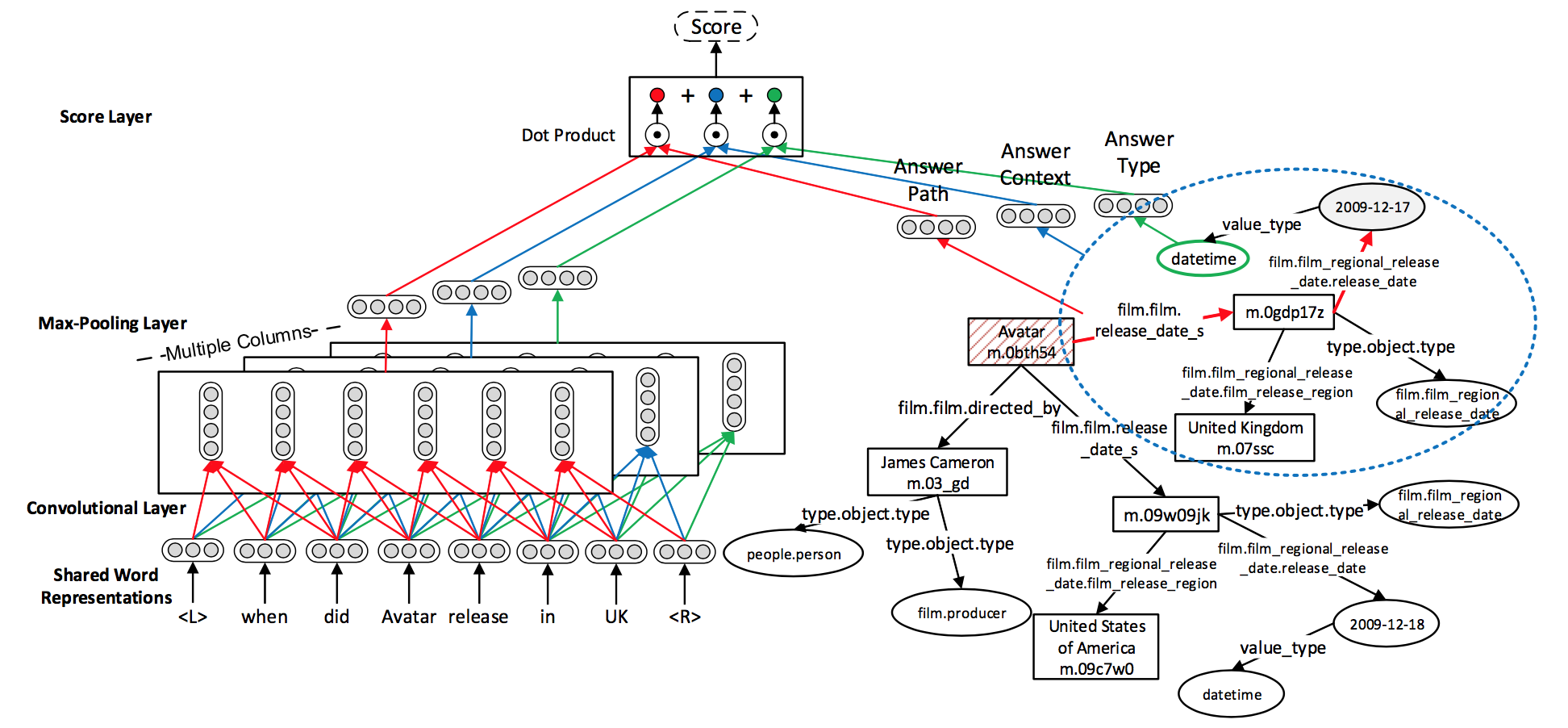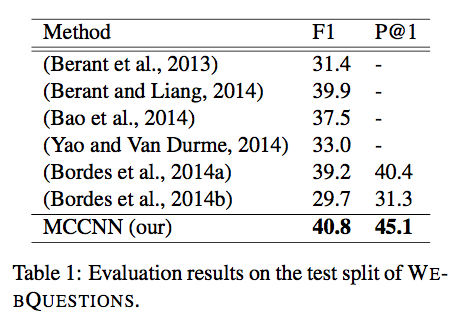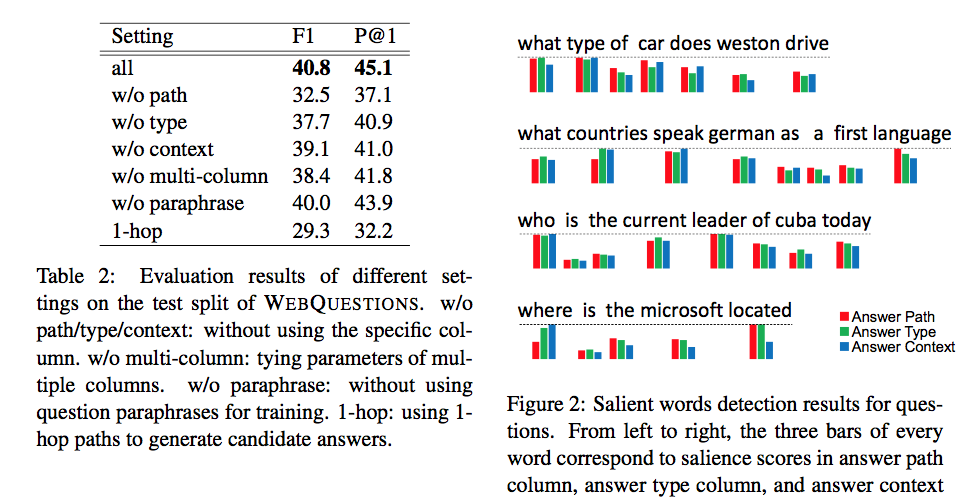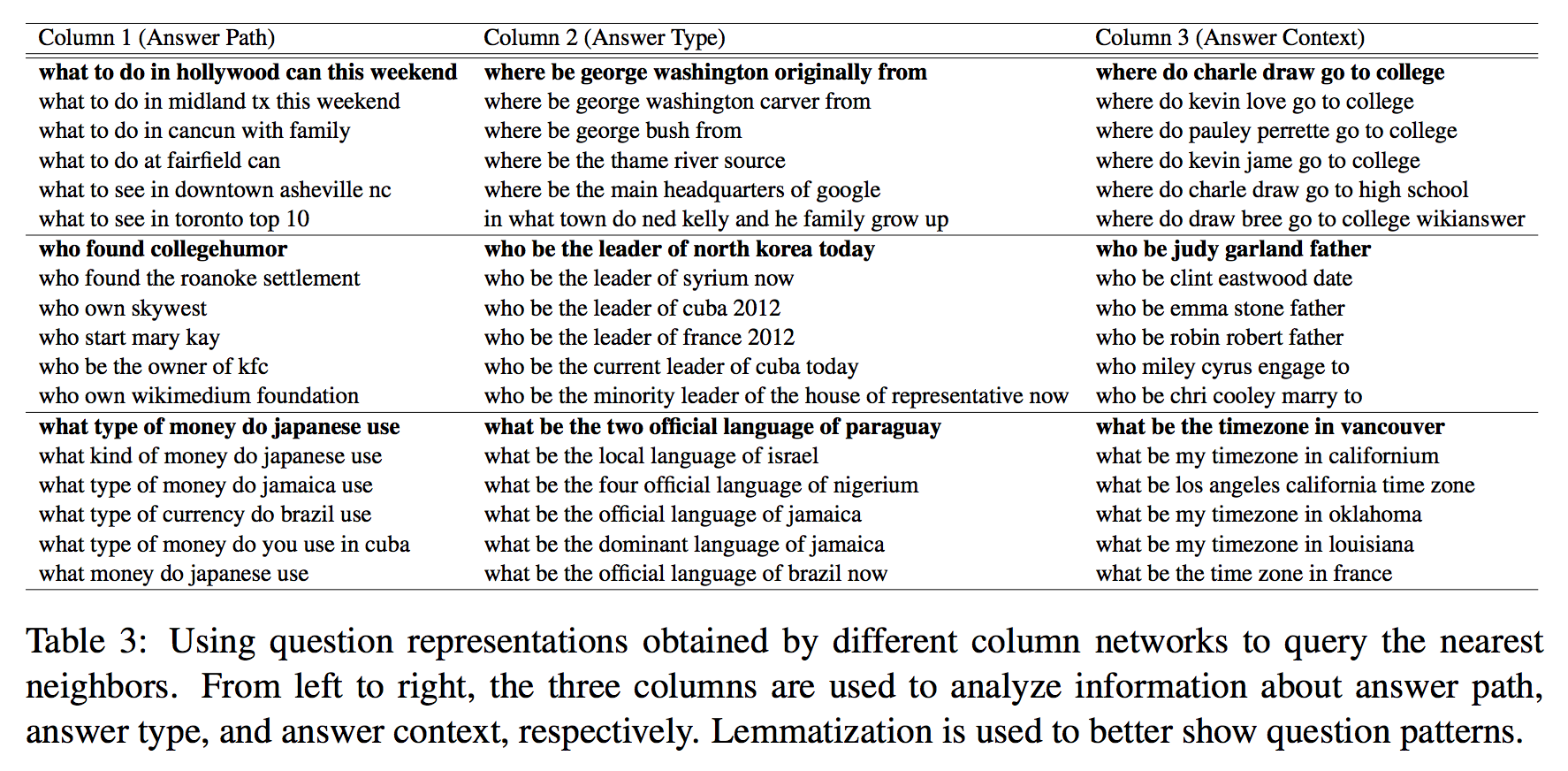# 【论文笔记】Question Answering over Freebase with Multi-Column Convolutional Neural Networks

2.1 模型简介
假设存在问题q=w1...wn$q = w_{1} . . . w_{n}$，作者使用 Freebase Search API来找到问题中的命名实体，如果问题中不含有任何命名实体或者名词，那么就使用返回最相关的一个命名实体，然后使用其在知识库中悬着候选答案，所有的2-hops 节点的实体和关系都视为候选答案，表示为Cq$C_{q}$
然后基于每一个候选答案a，根据模型训练得到的答案路径（answer path）, 答案背景信息（answer context）, 以及答案类型（answer type) 的vector representations，以及分别对应的问题的vector representations，即：f1(q)f2(q)f3(q)$f_{1}(q)，f_{2}(q)，f_{3}(q)$，最后根据S (q, a)才选出答案，计算方法为：

S(q,a)=f1(q)Tg1(a)+f2(q)Tg2(a)+f3(q)Tg3(a)$S (q, a) =f_{1}(q)^{T}g_{1}(a) + f_{2}(q)^{T}g_{2}(a) + f_{3}(q)^{T}g_{3}(a)$

其中fi(q)gi(a)$f_{i}(q)与g_{i}(a)$的维度相同，它们的计算方式为內积，最后把三项相加而得。2.2 MCCNNs for Question Understanding
MCCNNs使用了三个管道来学习问题表示和word embeddings，分别对应答案路径，答案背景信息, 以及答案类型。假设存在问题q=w1...wn$q = w_{1} . . . w_{n}$，把每个词使用lookup转为词向量，即：wj=Wvu(wj)$w_{j} = W_{v}u(w_{j})$WvRdv×|V|$W_{v} ∈ R^{d_{v}×|V |}$u(wj)$u(w_{j} ) ∈${0, 1}^|V|${|V|}$为词wj$w_{j}$的one-hot representation，|V|为词表大小。
那么对于MCCNNs的第i个通道，表示问题q的n个向量的第j个的计算方法为：

x(i)j=h(W(i)[wTjs...wTj...wTj+s]+b(i))$x^{(i)}_{j} =h(W_{(i)} [w^{T}_{j-s} ...w^{T}_{j}...w^{T}_{j+s}] +b^{(i)})$

fi(q)=maxj=1,...,n$f_{i} (q) = max_{j =1,...,n}${x(i)$\textbf{x}_{(i)}$}

对于每个候选答案a，其三方面的Vector representations分别表示为：g1(a),g2(a),g3(a)$g_{1}(a) , g_{2}(a) , g_{3}(a)$，其学习方式分别如下：
①Answer Path，答案路径表示为知识库中候选答案与问题中的实体节点之间路径上的所有关系（relation）集合，如上图的答案路径为：(film.film.release date s,film.film regional release date.release date)，其ector representation g1(a)$g_{1}(a)$计算方式为：
g1(a)=1||up(a)||1Wpup(a)$g_{1}(a) = \frac{1}{||u_{p}(a)||_{1}}\textbf{W}_{p}u_{p}(a)$

其中up(a)R|R|×1$u_{p}(a) ∈ R^{|R|×1}$为维度大小为|R|的向量，即如果在这个某个实体关系出现了，那么该位置值为1，否则为0，|R|表示关系的个数，WpRdq×|R|$W_{p} ∈ R^{d_{q}×|R|}$为权值矩阵，·1$∥·∥_{1}$为L1范数，所以这里最终的意思可以解释为所有出现的关系（relation）的embedding的平均。
g2(a)=1||uc(a)||1Wcuc(a)$g_{2}(a) = \frac{1}{||u_{c}(a)||_{1}}\textbf{W}_{c}u_{c}(a)$

其中uc(a)R|C|×1$u_{c}(a)∈ R^{|C|×1}$为维度大小为|R|的向量，即如果在这里某个实体或关系出现了，那么该位置值为1，否则为0，|C|表示在所有上下文中出现的实体和关系的个数，WcRdq×|C|$W_{c} ∈ R^{d_{q}×|C|}$为权值矩阵，·1$∥·∥_{1}$为L1范数。
g3(a)=1||ut(a)||1Wtut(a)$g_{3}(a) = \frac{1}{||u_{t}(a)||_{1}}\textbf{W}_{t}u_{t}(a)$

其中ut(a)R|T|×1$u_{t}(a)∈ R^{|T|×1}$为维度大小为|R|的向量，即如果在这个某个实体关系出现了，那么该位置值为1，否则为0，|T|表示在所有类型的种类个数，WtRdq×|T|$W_{t} ∈ R^{d_{q}×|T|}$为权值矩阵，·1$∥·∥_{1}$为L1范数。
2.4 Model Training
在训练集中，对于问题q的每一个正确答案aAq$a ∈ A_{q}$（一个问题的正确答案可能不止一个），随机在候选答案Cq$C_{q}$中选择k个错误的答案a$a^{'}$，其loss函数可以构造为：
l(q,a,a=(mS(q,a)+S(q,a)+$l(q,a,a^{'}） =(m−S(q,a)+S(q,a^{'})_{+}$

其中m为margin parameter，(z)+$(z)_{+}$= max{0, z}，那么最终的loss函数可以表示为：
minq1|Aq|aAqaRql(q,a,a$min\sum_{q}{\frac{1}{|A_{q}|}\sum_{a∈A_{q} }\sum_{a^{′}∈R_{q}}}l(q,a,a^{'}）$

其中|Aq|$|A_{q}|$表示每个问题正确答案的个数。
因为对于每一个问题，其对应的正确答案可能不止一个，所以设定了阈值m，即loss函数中的margin parameter，当通过下列公式来判别是否选为正确答案：
{Aq$A_{q}$={ a|aCq$a|a∈C_{q}$ and max{ S(q,a)−S(q,a) <m }上图左是作者基于学到的问题表示f(q)，计算问题中每个词的重要性，例如存在问题q=wn1$q = w_{1}^{n}$，其中第j个词wj$w_{j}$的重要性计算方法为：

ei(wj)=||fi(wn1)fi(w1wjwj)||2$e_{i}(w_{j}) = ||f_{i}(w_{1}^{n}) − f_{i}(w_{1}w_{j}^{'}w_{j})||_{2}$

其中wj$w_{j}^{'}$为替换掉wj$w_{j}$的词，常用is, to, a等停用词表示，从结果可以看出以wh-开头的词比较重要，另外依靠于wh-词汇的动词和名词也比较重要。下图是作者使用不同通道的问题表示f(q)，根据每个问题的余弦值计算相近问题得到的结果。